In :
import os
import numpy as np
import pandas as pd
from SimPEG import Mesh, Maps, DC, Utils
from pymatsolver import PardisoSolver
import matplotlib.pyplot as plt
from pylab import hist
from matplotlib import colors
%matplotlib inline


# Running 2D DC inversion using SimPEG¶

## Purpose¶

We demonstrate how 2D line of field DC data can be inverted in SimPEG. The field DC data is from Syscal Pro system and exported to csv format. Follwing eight steps will be illustrated to guide how 2D DC inversion can be run within SimPEG.

• Step1: Read in DC data in csv format
• Step2: Generate DC.IO object
• Step3: Generate Mapping
• Step4: Form Problem object for physics
• Step5: Set uncertainty for the data
• Step6: Set initial model
• Step7: Run inversion
• Step8: Plot results

## Step1: Read in DC data in csv format¶

Example file used here is 'dc_data.csv'. We first load this csv file using pandas then input survey information to DC.IO object.

### Read csv file using pandas¶

In :
# Downlaod an example DC data

fname = os.path.sep.join(["..", "data", "dc_data.csv"])

In :
# file name
header_loc = ['Spa.'+str(i+1) for i in range(4)]
# Apparent resistivity

In :
df.describe()

Out:
Unnamed: 0 Spa.1 Spa.2 Spa.3 Spa.4 Rho Dev. M Sp Vp ... Channel Overload Tx-Bat Rx-Bat Temp. Gapfiller Synch Cole Tau Cole M Cole rms
count 0.0 1211.000000 1211.000000 1211.000000 1211.000000 1211.000000 1211.000000 1211.000000 1211.000000 1211.000000 ... 1211.000000 1211.0 1211.000000 1211.000000 1211.000000 1211.0 1211.000000 1211.0 1211.0 1211.0
mean NaN 214.219653 219.219653 255.780347 260.780347 181.833691 42.542543 34.878596 0.084979 -108.493249 ... 4.620149 0.0 11.688489 11.828159 46.093064 0.0 0.145334 0.0 0.0 0.0
std NaN 125.705571 125.705571 125.705571 125.705571 157.068458 104.394421 146.461954 142.643142 242.930941 ... 2.836490 0.0 0.109590 0.051543 0.667536 0.0 0.352583 0.0 0.0 0.0
min NaN 0.000000 5.000000 10.000000 15.000000 -12.080000 0.000000 -163.520000 -549.960000 -2397.696000 ... 1.000000 0.0 11.470000 11.620000 44.600000 0.0 0.000000 0.0 0.0 0.0
25% NaN 105.000000 110.000000 150.000000 155.000000 71.250000 0.520000 0.175000 -39.945000 -75.698000 ... 2.000000 0.0 11.590000 11.800000 45.600000 0.0 0.000000 0.0 0.0 0.0
50% NaN 215.000000 220.000000 255.000000 260.000000 139.170000 4.820000 2.030000 -0.910000 -11.334000 ... 4.000000 0.0 11.710000 11.850000 46.100000 0.0 0.000000 0.0 0.0 0.0
75% NaN 320.000000 325.000000 365.000000 370.000000 254.965000 25.470000 7.965000 38.005000 -2.512500 ... 7.000000 0.0 11.800000 11.870000 46.500000 0.0 0.000000 0.0 0.0 0.0
max NaN 460.000000 465.000000 470.000000 475.000000 1107.340000 816.490000 999.990000 528.160000 0.143000 ... 10.000000 0.0 11.850000 11.920000 48.000000 0.0 1.000000 0.0 0.0 0.0

8 rows × 78 columns

There are a number of columns in the pandas' data frame, df. Here we only select ABMN locations and the apparent resistivity, which will be input variabls of DC.IO object.

In :
# Number of the data
# ABMN locations
# Apparent resistivity


## Step2: Generate DC.IO object¶

DC.IO will enable us to form DC survey and Mesh which are required information to run DC simulation. Survey information that we have selected are required to be input to DC.IO. Based upon the survey information, 2D mesh will be generated.

In :
# DC.IO object
IO = DC.IO()
# Generate DC survey using IO object
dc_survey = IO.from_ambn_locations_to_survey(
a, b, m, n,
survey_type='dipole-dipole',
data_dc=apprho,
data_dc_type='apparent_resistivity'
)

/Users/lindseyjh/git/python_symlinks/SimPEG/EM/Static/DC/IODC.py:228: UserWarning: code under construction - API might change in the future
"code under construction - API might change in the future"

In :
IO.plotPseudoSection(data_type='apparent_resistivity', scale='linear')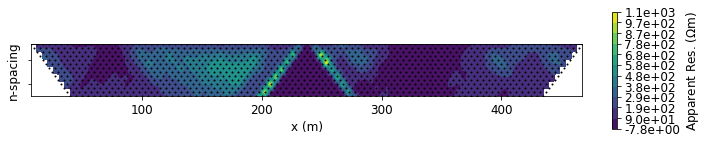In :
# Generate 2D tensor mesh
mesh, actind = IO.set_mesh(dx=2.5)

/Users/lindseyjh/git/python_symlinks/SimPEG/EM/Static/DC/IODC.py:505: UserWarning: dz (1.25 m) is set to dx (2.5 m) / 2
"dz ({} m) is set to dx ({} m) / {}".format(dz, dx, 2)

In :
fig, ax = plt.subplots(1,1,figsize=(10, 5))
mesh.plotGrid(ax=ax)
plt.gca().set_aspect(4)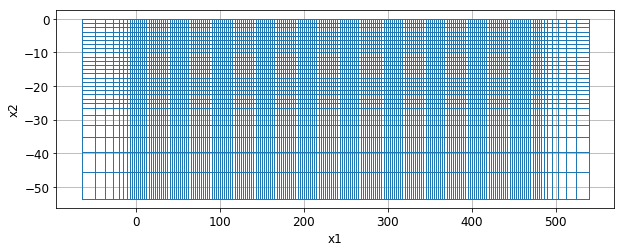## Step3: Generate Mapping¶

When inverting DC data often inversion model, $m$, differ from the conductivity, $\sigma$. For instance, often logarithmic conductivity is used as an inversion model:

$$m = log (\sigma)$$

$$\sigma = \mathcal{M} (m)$$

Thus, the mapping, $\mathcal{M} (m)$ transform from a model space to a physical property space. Here we use exponential map:

$$\sigma = exp(m)$$

which is equivalent to logarithmic conductivity.

In :
# Exponential map
sigmaMap = Maps.ExpMap(mesh)

In :
print (sigmaMap * np.ones(mesh.nC) * np.log(1./100.))

[-12.51815043 -12.51815043 -12.51815043 ... -12.51815043 -12.51815043
-12.51815043]


## Step4: Form Problem object for physics¶

Problem object has a capability to evaluate predicted data, $d^{pred}=F[m]$, for a given model, $m$. Survey information and Mapping are required to evaluate the predicted data. Here we use DC.Problem2D_N. Potentials are defined on the nodes and 2.5D formulation is used.

In :
# Problem ojbect
prob = DC.Problem2D_N(
mesh, sigmaMap=sigmaMap, storeJ=True,
Solver=PardisoSolver
)
if prob.pair:
prob.unpair()
if dc_survey.pair:
dc_survey.unpair()
# Paring survey and problem
prob.pair(dc_survey)


## Step5: Set uncertainty for the data¶

A minimal condition for the DC inversion is making sure finding a model that fits the observed data. Data misfit is often defined as

$$\phi_d = \Sigma_{i}^{N}\Big(\frac{d^{pred}_i-d^{obs}_i}{\varepsilon_i}\Big)^2$$

,

where $\varepsilon_i$ is the uncertainty at $i$-th dataum. We often assign percentage ($\%$) and floor as uncertainty, which can be written as:

$$\varepsilon_i = \% \ |d^{obs}_i| + floor$$

Note that the unit of the data is volatage (V) and hence apparent resistivity need to be converted to voltage.

In :
out = hist(np.log10(abs(IO.voltages)+1e-4), bins=100)
plt.xlabel("Log 10 voltage (V)")

Out:
Text(0.5, 0, 'Log 10 voltage (V)')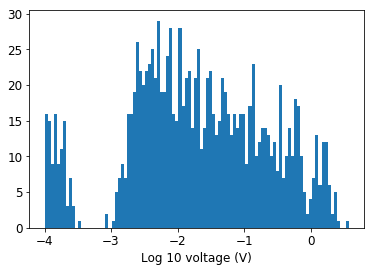In :
# Set the observed data as the measured voltage
dc_survey.dobs = IO.voltages.copy()
# Set percentage as 5%
std = 0.05
# Set floor as 1e-3 V
eps = 1e-3


## Step6: Set initial model¶

For any gradient-based inversion setting a reasonable initial model is very important. Here we set an initial model based upon the observed apparent resistivity values.

In :
out = hist(np.log10(abs(IO.apparent_resistivity)+1e-1), bins=100)
plt.xlabel("Log 10 apparent resistivity")

Out:
Text(0.5, 0, 'Log 10 apparent resistivity')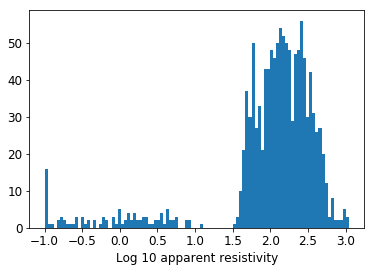In :
m0 = np.ones(mesh.nC) * np.log(1./100.)


## Step7: Run inversion¶

In order to run DC inversion, multiple ojbects are required:

• DataMifit: Data misfit L2
• Regularization: Tikhonov Style regularization
• Optimization: Projected Gauss Newtown
• InvProblem: Statement of the inversion
• Directives: Conductor of the inversion
• Inversion: Running inversion
In :
from SimPEG import (DataMisfit, Regularization,
Optimization, Inversion, InvProblem, Directives)

## DataMisfit (L2)
dmisfit = DataMisfit.l2_DataMisfit(dc_survey)
uncert = abs(dc_survey.dobs.copy()) * std + eps
dmisfit.W = 1./uncert

## Regularization
# Map for a regularization
regmap = Maps.IdentityMap(nP=int(actind.sum()))
reg = Regularization.Simple(mesh, indActive=actind, mapping=regmap)
reg.alpha_s = 1.
reg.alpha_x = 1.
reg.alpha_y = 1.
# This is for sparse inversion
# reg = Regularization.Sparse(mesh, mapping=regmap)
# reg.norms = np.c_[0., 2., 1., 1.]
# IRLS = Directives.Update_IRLS(maxIRLSiter=15, minGNiter=1)

## Optimization
maxIter = 10
upper = np.log(1e1)
lower = np.log(1e-4)
opt = Optimization.ProjectedGNCG(maxIter=maxIter, upper=upper, lower=lower)

## InvProblem
invProb = InvProblem.BaseInvProblem(dmisfit, reg, opt)

## Directives
# 1: Initial beta estimation
beta0_ratio = 1.
betaest = Directives.BetaEstimate_ByEig(beta0_ratio=beta0_ratio)
# 2: Beta cooling schedule
coolingFactor = 2.
coolingRate = 1
beta = Directives.BetaSchedule(
coolingFactor=coolingFactor, coolingRate=coolingRate
)
# 2: Target misfit
target = Directives.TargetMisfit()
# 4: Save outputs
save = Directives.SaveOutputEveryIteration()
directiveList = [
beta, betaest, save, target
]
# This is for Sparse inversion
#         directiveList = [
#             beta, betaest, IRLS, save
#         ]

## Inversion
inv = Inversion.BaseInversion(
invProb, directiveList=directiveList,
)

SimPEG.DataMisfit.l2_DataMisfit assigning default std of 5%
SimPEG.DataMisfit.l2_DataMisfit assigning default eps of 1e-5 * ||dobs||

In :
# Run inversion
mopt = inv.run(m0)

SimPEG.InvProblem will set Regularization.mref to m0.

SimPEG.InvProblem is setting bfgsH0 to the inverse of the eval2Deriv.
***Done using same Solver and solverOpts as the problem***
Compute fields
SimPEG.SaveOutputEveryIteration will save your inversion progress as: '###-InversionModel-2019-01-26-16-17.txt'
model has any nan: 0
=============================== Projected GNCG ===============================
#     beta     phi_d     phi_m       f      |proj(x-g)-x|  LS    Comment
-----------------------------------------------------------------------------
x0 has any nan: 0
Compute fields
0  9.18e+01  9.01e+05  0.00e+00  9.01e+05    3.02e+02      0
Compute fields
1  4.59e+01  8.59e+04  5.55e+01  8.85e+04    2.55e+02      0
Compute fields
2  2.30e+01  2.36e+04  1.75e+02  2.76e+04    2.18e+02      0   Skip BFGS
Compute fields
3  1.15e+01  1.31e+04  3.47e+02  1.71e+04    1.88e+02      0   Skip BFGS
Compute fields
4  5.74e+00  6.55e+03  6.60e+02  1.03e+04    1.57e+02      0   Skip BFGS
Compute fields
5  2.87e+00  2.40e+03  1.08e+03  5.48e+03    1.27e+02      0   Skip BFGS
Compute fields
6  1.43e+00  1.02e+03  1.37e+03  2.98e+03    8.65e+01      0   Skip BFGS
Compute fields
------------------------- STOP! -------------------------
1 : |fc-fOld| = 0.0000e+00 <= tolF*(1+|f0|) = 9.0073e+04
1 : |xc-x_last| = 4.1752e+00 <= tolX*(1+|x0|) = 3.4777e+01
0 : |proj(x-g)-x|    = 8.5321e+01 <= tolG          = 1.0000e-01
0 : |proj(x-g)-x|    = 8.5321e+01 <= 1e3*eps       = 1.0000e-02
0 : maxIter   =      10    <= iter          =      7
------------------------- DONE! -------------------------


## Step 8: Plot results¶

Here we plot results of the DC inversion.

• Recovered 2D conductivity model
• Observed and Predicted data
• Tikhnov curves

### Recovered conductivity model¶

In :
# Transform log conductivity model to conductivity
sigma_est = sigmaMap * mopt

In :
# Extract Core Mesh and corresponding indices
actind_core, mesh_core = Utils.ExtractCoreMesh(IO.xyzlim, mesh)

In :
mesh_core.plotImage(sigma_est[actind_core], pcolorOpts={'norm':colors.LogNorm(), 'cmap':'jet'}, clim=(1e-3, 1e-1))
plt.title("Recovered conductivity")
plt.gca().set_aspect(4)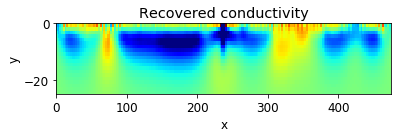### Observed and Predicted data¶

In :
plt.semilogy(abs(dc_survey.dobs))
plt.semilogy(abs(invProb.dpred))
plt.legend(("Observed", "Predicted"))

Out:
<matplotlib.legend.Legend at 0x137f98cc0>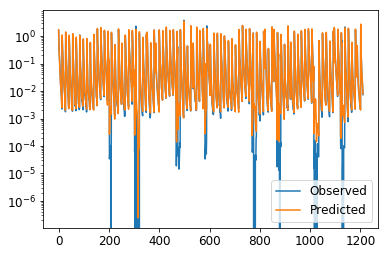### Tikhnov curves¶

In :
save.plot_tikhonov_curves()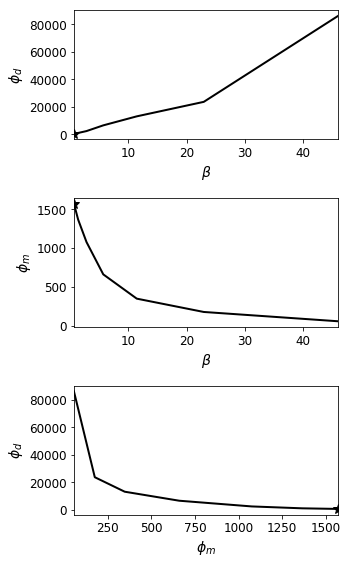In [ ]: